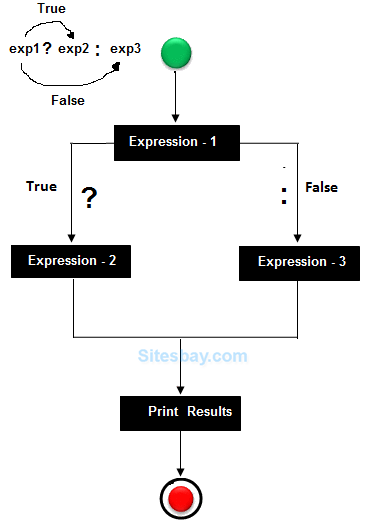# Find Greatest Number Program in C

## Find Largest Number Program in C

```#include<stdio.h>
#include<conio.h>

void main()
{
int a,b,c;
clrscr();
printf("Enter any three number: ");
scanf("%d%d%d",&a,&b,&c);

if(a >= b && a >= c)
printf("%d is largest",a);
else if(b >= a && b >= c)
printf("%d is largest",b);
else if(c >= a && c >= b)
printf("%d is largest",c);
getch();
}
```

## Output

```Enter any three numbers:
5
10
4
10 is largest
```

## Ternary operator

If any operator is used on three operands or variable is known as ternary operator. It can be represented with " ?: "In the above symbol exp1 is condition and exp2 and exp3 will be either value or variable or statement or any mathematical expression. If condition will be true exp2 will be execute otherwise exp3 will be executed.

## Find Largest Number Program in C

```#include<stdio.h>
#include<conio.h>

void main()
{
int a,b,c,largest;
clrscr();
printf("Enter any three numbers: ");
scanf("%d%d%d",&a,&b,&c);
largest=(a>b)?(a>c?a:c):(b>c?b:c);
printf("Largest number:  %d",largest);
getch();
}
```

## Output

```Enter any three numbers:
5
10
4
Largest number: 10
```

WIFI CCTV Camera